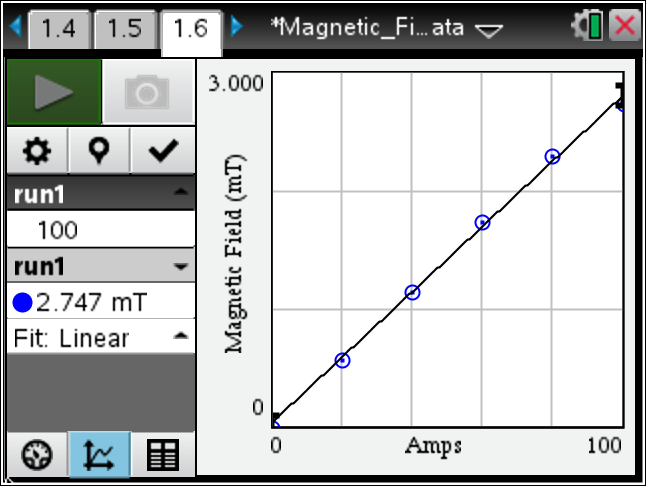# Activities

•##### Download

•• ##### Subject Area

• Science: Physics: Electrostatics

• ##### Author9-12

45 Minutes

• ##### Device
•TI-Nspire™ CX/CX II
•TI-Nspire™ CX CAS/CX II CAS
• TI-Nspire™ Navigator™

3.6

## Magnetic Field of a Coil - Data Collection#### Activity Overview

In this lesson, students will explore the proportional relationship between the magnetic flux density at the center of a long, electrically charged coil and the strength of the electrical current.

#### Objectives

• Students will understand the relationship between magnetic flux density and the strength of the electrical current.
• Students will determine the value of the magnetic constant.

#### Vocabulary

• Magnetic flux density
• Electric Current

#### About the Lesson

This lesson demonstrates the proportional relationship between the magnetic flux density at the center of a long, electrically charged coil and the strength of the electrical current. This is a more advanced experiment for high school students.
As a result, students will:

• Create a closed circuit.
• Determine a relationship from a graph.
• Calculate the value for the magnetic constant.
• Calculate percent error of the experimental value from the given true value.